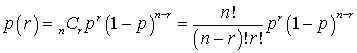### Why should I choose AnalystNotes?

AnalystNotes specializes in helping candidates pass. Period.

##### Subject 5. Binomial Distribution
When a coin is flipped, the outcome is either heads or tails. When a magician guesses the card selected from a deck, the magician can either be correct or incorrect. When a baby is born, the baby is either born in the month of March or is not. In each of these examples, an event has two mutually exclusive possible outcomes. For convenience, one of the outcomes can be labeled "success" and the other outcome "failure." If an event occurs N times (for example, a coin is flipped N times), then the binomial distribution can be used to determine the probability of obtaining exactly r successes in the N outcomes.

A Bernoulli trial is an experiment with two outcomes, which can represent success or failure, up move or down move, or another binary outcome. As one of these two outcomes must definitely occur, that is, they are exhaustive, and also mutually exclusive, it follows immediately that the sum of the probabilities of a "success" and a "failure" is 1.

A binomial random variable X is defined as the number of successes in n Bernoulli trials. The assumptions are:

• The probability (p) of success is constant for all trials. Similarly, the failure probability 1 - p stays constant throughout the experiment.
• The trials are independent. Thus, the outcome of one trial does not in any way affect the outcome of any subsequent trial.
• The sampling is done with replacement. This means that once an outcome has occurred, it is not precluded from occurring again.

The binomial probability for obtaining r successes in n trials is:where p(r) is the probability of exactly r successes, n is the number of events, and p is the probability of success on any one trial. This formula assumes that the events are:

• dichotomous (fall into only two categories)
• mutually exclusive
• independent
• randomly selected

To remember the formula, note that there are three components:

• n!/[(n-r)! x r!]. This indicates the number of ways r successes can be achieved and n - r failures in n trials, where the order of success or failure does not matter. This is the combination formula.
• pr. This is the probability of getting r consecutive success.
• (1 - p)n-r. This is the probability of getting n - r consecutive failures.

The values for n and p will always be given to you in a question; their values will never have to be guessed.

Consider this simple application of the binomial distribution. What is the probability of obtaining exactly 3 heads if a coin is flipped 6 times? For this problem, n = 6, r = 3, and p = 0.5, =>p(3) = {6!/[(6 - 3)! x 3!]}0.53(1 - 0.5)6-3 = 0.3125.

Often the cumulative form of the binomial distribution is used. To determine the probability of obtaining 3 or more successes with n = 6 and p = 0.3, compute p(3) + p(4) + p(5) + p(6).

For a single Bernoulli random variable, Y, which takes on the value 1 with probability p and the value 0 with probability 1 - p, the mean is p and the variance is p(1 - p).

Every random variable has a mean and a variance associated with it. A general binomial random variable, B(n, p), is the sum of n Bernoulli random variables, and so the mean of a B(n, p) random variable is np. Given that a B(1, p) variable has variance p(1 - p), the variance of a B(n, p) random variable is n times that value, or np(1 - p), using the independent assumption.

For example, for a B(n = 5, p = 0.10) random variable, the expected number of successes is 5 x 0.1 = 0.5 with a standard deviation of (5 x 0.1 x 0.9)1/2 = 0.67.

Learning Outcome Statements

e. define the properties of a Bernoulli random variable and a binomial random variable, and calculate and interpret probabilities given the binomial distribution function;

CFA® 2022 Level I Curriculum, Volume 1, Module 4

### User Contributed Comments13

User Comment
Natalia82 Anecdote:

A man and a woman were asked the same question:
"What is the probability of running into a dinosaur in the street?"

M: 0! It's impossible!
W: 1/2! I will either meet him or not.
dah62 Natalia: You've written the male response wrong as 0! is 1 therefore 100% certain, not impossible as the second statement says. ;-)
Seemorr Very nice dah62
Vikku One can only get philosophical after reading these notes. All the best to CFA aspirants!
thammy Ha ha ha ha...Thank you guys for making this CFA process so much more bearable.. I would highly recommend this site not only because of the quality of their contents but also because of user comments. :)
Sego I agree. You guys just rock
hardig in the example at the end of this, where did p=0.5 come from? I am assuming it is 1/2 from the standpoint that heads is 1 of 2 possible results of flipping the coin, but just want to confirm....
Saxonomy hardig, the expected value of B(n,p) is simply n multiplied by p. So in the example, the exp value of B(5, .1) is 5 x 0.1 = 0.5

B(n,p) means n variable a.k.a. trials, with each variable/trial having a p% chance of being correct.

Now back to the humor, family!
gtt240 I don't understand where the .9 came from here at the end when calculating the standard deviation.
abrown3474 gtt240- That is the (1-p) portion of the equation. In this case, 1-.10=.90
birdperson for the 3 coin flip example, here is how you can do it on your BAII
--> use nCR --> 6C3 * (0.5)^3 *(0.5)^3
Chl4072 Bird person, how to key in BA II. ..?
akhlo 2nd "+"